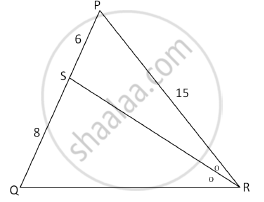# In the following figure, in ΔPQR, seg RS is the bisector of ∠PRQ. If PS = 6, SQ = 8, PR = 15, find QR. - Geometry

In the following figure, in ΔPQR, seg RS is the bisector of ∠PRQ. If PS = 6, SQ = 8, PR = 15, find QR.#### Solution

Seg RS bisects ∠PRQ ….(given)

(PR)/(QR)= (PS)/(SQ) ....(angle bisector property)

15/(QR)=6/8

QR= (15xx8)/6=20

Concept: Similarity of Triangles
Is there an error in this question or solution?